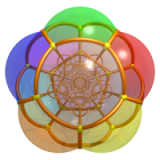# 楔子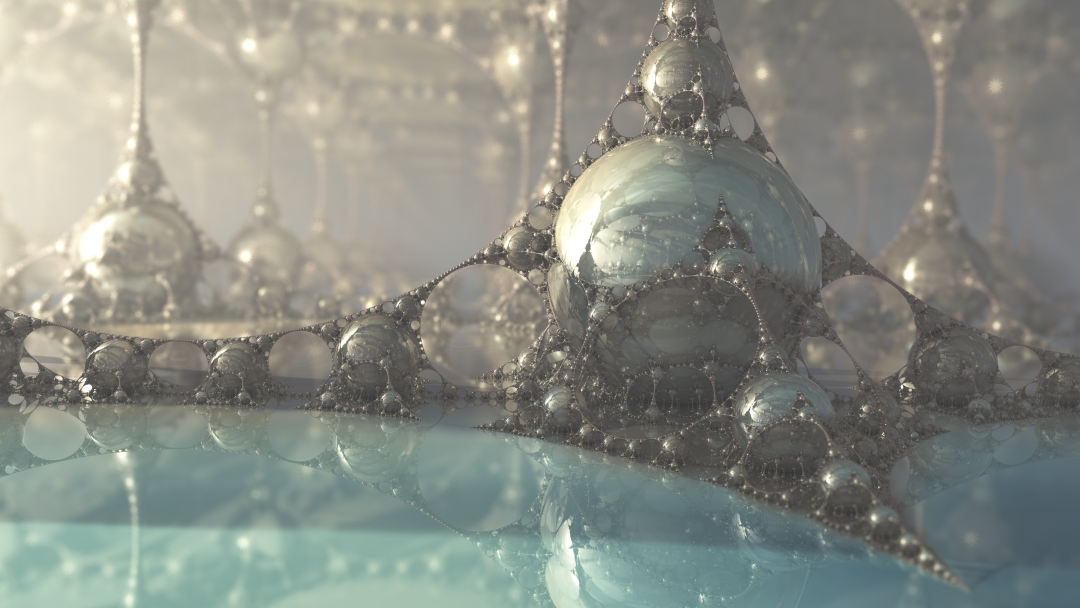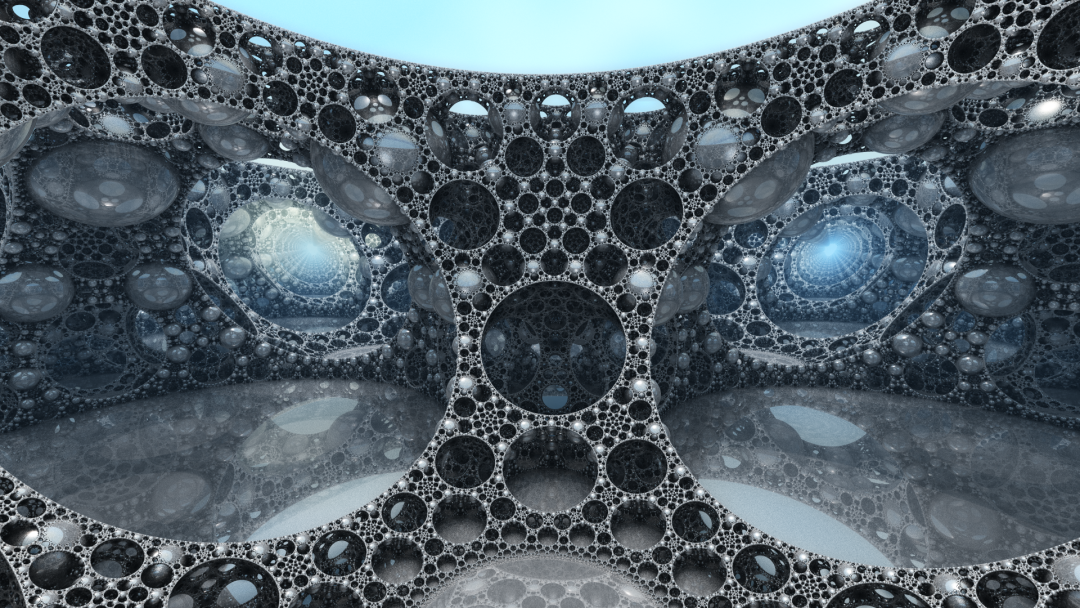# 万花筒是关于镜子的魔术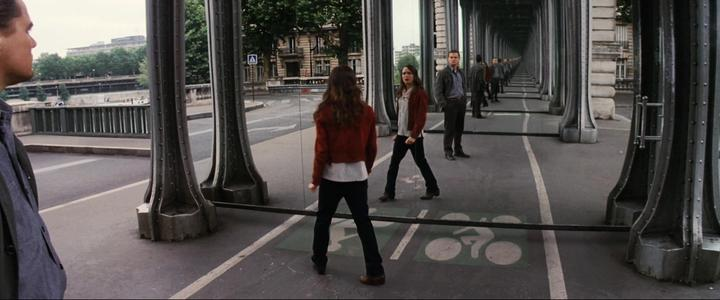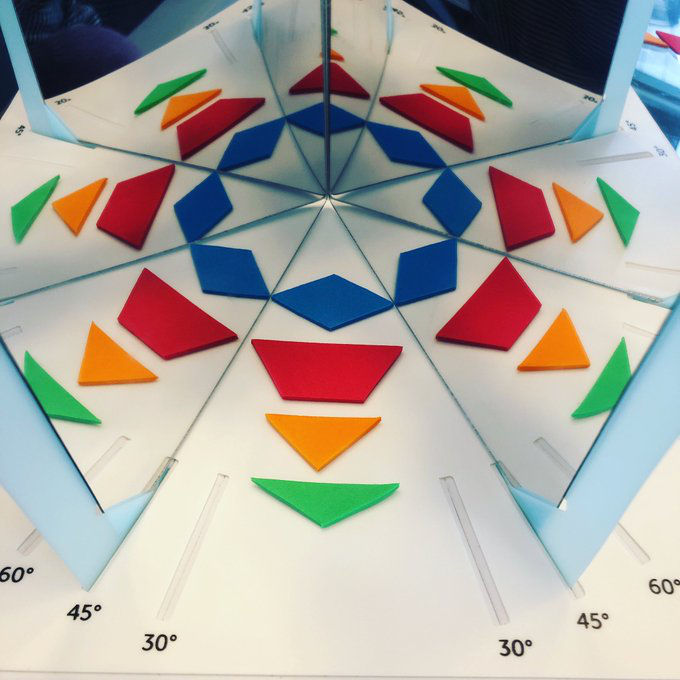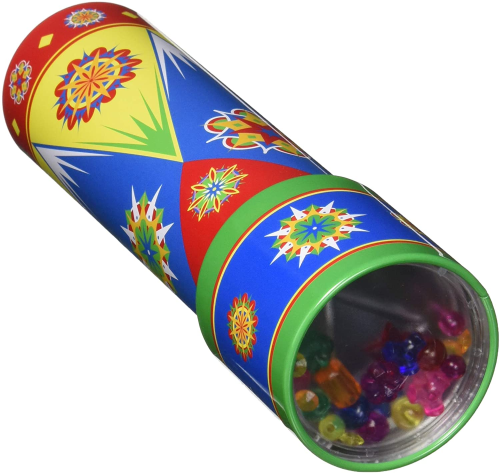# 万花筒的对称群

1. 恒等变换 $1\in W$
2. $g,g'\in W$ 是两个变换，则它们的复合 $gg'\in W$
3. $g\in W$，则 $g$ 的逆变换 $g^{-1}\in W$

$W$ 叫做万花筒的对称群，$S$ 中的元素叫做 $W$ 的生成元。

$m=\infty$ 时，我们认为 $\rho_i$$\rho_j$ 之间没有任何关系。

1. $M$ 的对角元都是 2。
2. $M$$(i,j)$ 分量 $m_{ij}$ 表示对 $s_i,s_j\in S$$(s_is_j)^{m_{ij}}=1$ 成立。

$s_i$ 对应的就是万花筒中关于镜面 $i$ 的单反射 $\rho_i$，这里抽象 Coxeter 群的含义是，我们抛弃了每个 $\rho_i$ 的具体表现形式，而仅考虑它们之间满足的结构关系。对一个抽象 Coxeter 群 $W$，可能有不同的万花筒都以 $W$ 为对称群，这时 $s_i$ 作为反射 $\rho_i$ 有不同的实现形式。

1. 当两个镜面互相垂直时，它们之间没有边相连。
2. 当两个镜面夹角恰好是 $\pi-\pi/3$ 时，它俩用一条无标记的边相连。
3. 当两个镜面夹角是 $\pi-\pi/m$$m\in\{4,5,\ldots\}$ 时用一条标记为 $m$ 的边相连。
4. 两个平行的镜面用一条标记为 $a$ 的边相连，这里 $a$ 是一个 $\leq-1$ 的实数。当 $a=-1$ 时这个记号也写作 $\infty$

# 欧氏万花筒1. 一维的欧氏万花筒是在二维空间中处理的，镜面反射都是线性变换。
2. 基本区域 $\mathcal{D}$ 是一个无限大的锥形区域。
3. $\overline{\mathcal{D}}$ 的虚像填满了整个上半空间 $y > 0$ 和原点组成的锥形区域 (Tits 锥)。
4. 万花筒图案位于截面 $y=1$ 上。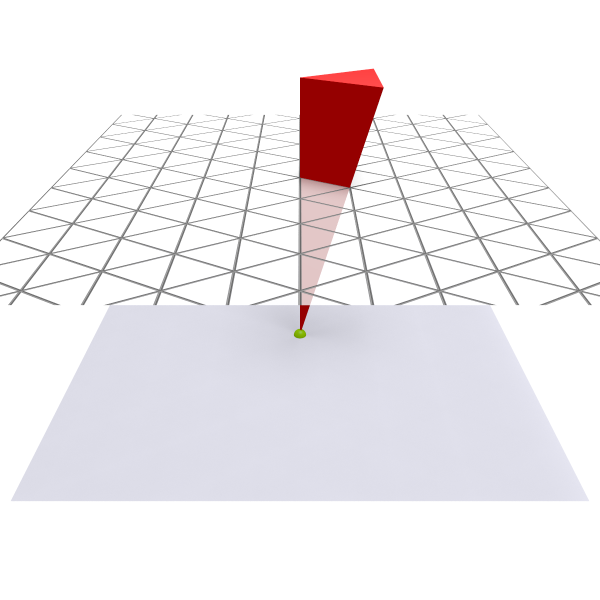$\widetilde{A}_2$ $\widetilde{B}_2$ $\widetilde{C}_2$原像在基本区域边上 原像在基本区域顶点原像在基本区域内部 原像在基本区域内部$\widetilde{A}_3$ $\widetilde{B}_3$ $\widetilde{C}_3$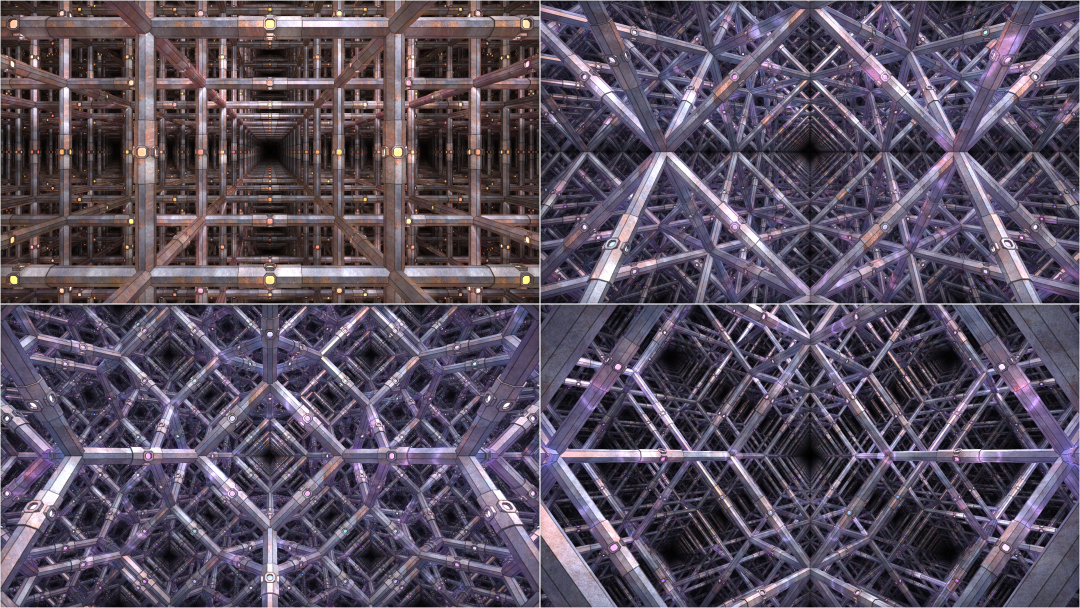：你可能要问了，如果我取正方体的六个面所在的平面为镜子，这样不就得到了一个有六面镜子的三维万花筒了吗？这种情形下镜面可以分为三组平行镜面，每组内的两个镜面是平行的，不同组之间的镜子是互相垂直的，这个万花筒本质是三个一维万花筒的“直积”，即不同组的镜面产生的虚像互不干涉，我们称它是可约的

$n\geq4$ 维的欧氏万花筒我们没法直接看到，是不是可以把它投影到二维或者三维空间中来观察呢？不幸的是由于仿射 Coxeter 群只有固定的几种，这种投影并不会给出新的二维/三维欧氏万花筒，但是对某些合适的超平面，通过选取高维万花筒的一部分投影到超平面上，会得到所谓的准晶 (quasicrystals) 结构，比如通过将五维空间中的正方体网格 $\mathbb{Z}^5$ 的一部分投影到由五次单位根 $\zeta$ 给出的二维平面 $(1,\zeta,\zeta^2,\zeta^3,\zeta^4)$ 上(取这个复向量的实部和虚部张成的二维子空间)，可以得到著名的彭罗斯密铺：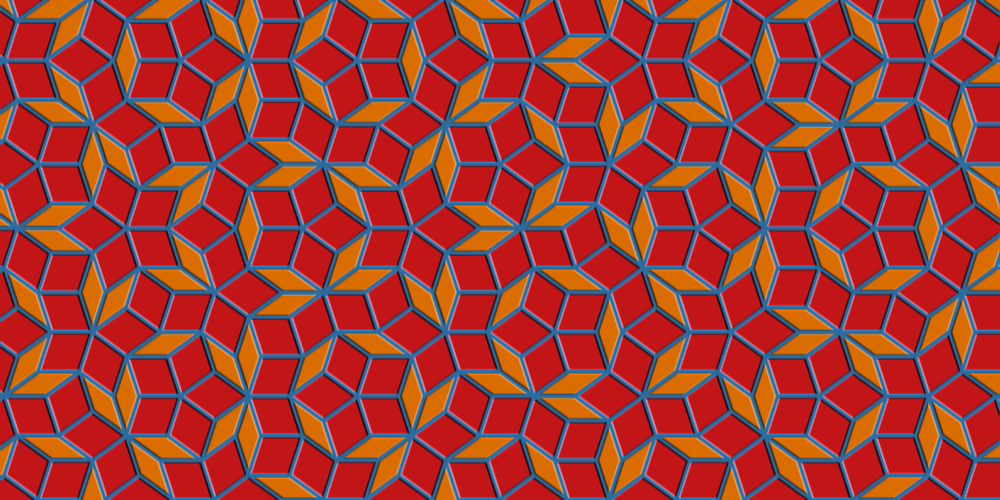# 球面万花筒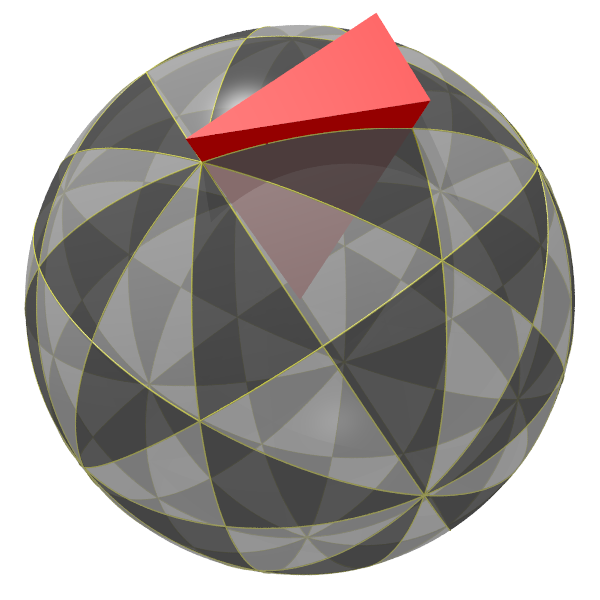1. 二维的球面万花筒是在三维空间中处理的。
2. 基本区域 $\mathcal{D}$ 是一个无限大的锥形区域。
3. $\overline{\mathcal{D}}$ 及其虚像填满了整个 $\mathbb{R}^3$
4. 万花筒图案位于截面 $x^2+y^2+z^2=1$ 上。

$A_3$ $B_3$ $H_3$ $I_m\times\mathbb{Z}_2$

Coxeter 图up 主使用的不是三面镜子哎，你能看懂成像的过程吗? 3

# 镜子必须是平的吗？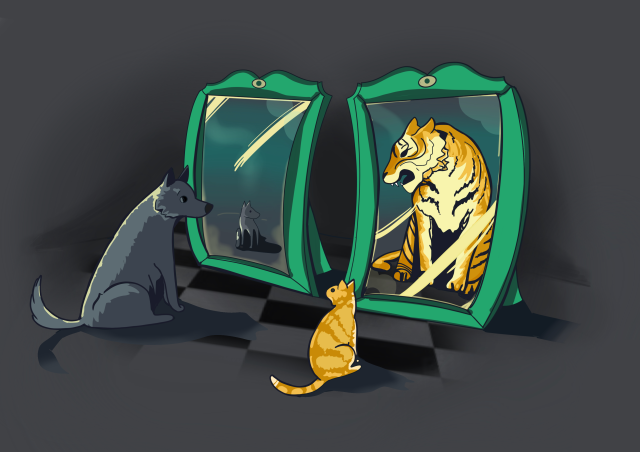$B(O, r)$ 是以空间中一点 $O$ 为中心，半径为 $r$ 的球，$P$ 是空间中任意一点。点 $P$ 关于球 $B(O,r)$ 的反演点 $P'$ 是射线 $\overrightarrow{OP}$ 上满足 $|OP|\cdot |OP'| = r^2$ 的唯一点。显然 $P'$ 关于 $B(O,r)$ 的反演点是 $P$，我们称 $P,P'$ 关于 $B(O,r)$ 互为反演点。规定球心 $O$ 和无穷远点互为反演点。$P$ 在球外时，可以这样计算反演点 $P'$：过 $P$ 作球 $B(O, r)$ 的任一切线，设切点为 $Q$，然后由 $Q$ 点向线段 $\overline{OP}$ 引垂线，垂足 $P'$ 即为所求的反演点。1. 它将空间中的任何球面/超平面仍然映射为球面/超平面。如果把超平面看作是半径无穷大的球面的话，那么这句话就可以简写为反演变换将球面映射为球面。
2. 它是保角的，即两条曲线 $\gamma(t),\gamma'(t)$ 在一点 $p$ 处的夹角等于变换后 $f(\gamma(t)),f(\gamma'(t))$$f(p)$ 处的夹角。
3. 一个球面/超平面在反演变换下被映射为自身，当且仅当其与反演球是正交的。

$S_n=\{(x_1,x_2,\ldots,x_{n+1})\,|\, x_1^2+x_2^2+\cdots+x_{n+1}^2=1\}$$\mathbb{R}^{n+1}$ 中的单位球面，把 $\mathbb{R}^n$ 等同于超平面 $x_{n+1}=0$，球极投影按照如下方式建立 $S_n$$\mathbb{R}^n\cup\{\infty\}$ 之间的一一对应：取 $q=(0,0,\ldots,1)$$S_n$ 上的北极点，对球面 $S_n$ 上任意一点 $p$，作射线 $\overrightarrow{qp}$ 与平面 $x_{n+1}=0$ 交于一点 $\widetilde{p}$$\widetilde{p}$ 即为 $p$ 点在球极投影下的对应点。此外规定 $q$ 的对应点为无穷远点。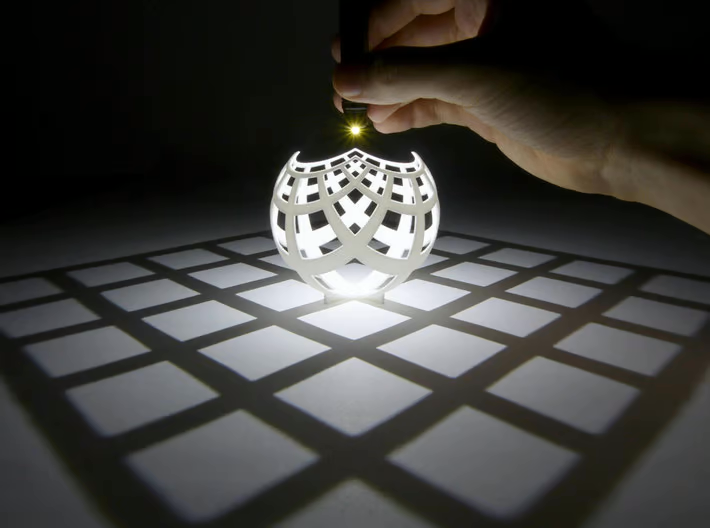Q：反演变换的原像和虚像大小不一致，这也能用在万花筒里吗？

Q$n$ 维的仿射变换可以放到 $n+1$ 维的空间中处理成线性变换，反演变换也可以吗？

# 二维 Poincaré 双曲空间1. 过直线 $l$ 外一点 $p$ 可以作无穷多条直线与 $l$ 平行。这里的直线应理解为双曲度量下的最短路径，即测地线：左边的这组直线与 $l$ 实际上是超平行的，它们与 $l$ 既不相交也不相切，但是它们与 $l$ 所夹的角度是不一样的。所以在双曲空间中平行这个概念不再简单地等价于“两条直线夹角为 0/180 度”。在双曲空间里面我们实际上可以用一个实数 $a$ 来标记两条直线的夹角：当两条直线相交时，$a$ 就是二者夹角的余弦 $a=\cos\theta\in(-1,1)$；若两条直线对应的圆相切，这时它们平行但不超平行，$a=\pm1$，其中 $a=+1$ 对应的是两圆内切，$a=-1$ 对应的是两圆外切；当两条直线超平行时 $|a|>1$，其中 $a>1$ 对应的是一个圆包含另一个，$a<-1$ 对应的是两圆不相交。这个实数 $a$ 叫做两个圆的 separation，它的具体计算方式我们暂时先不做介绍。

2. 任何三角形的内角和小于 180 度，最小可以是 0，最大可以任意接近 180 度：注意最外侧的三角形的三条边两两夹角都是 0，它们是双曲空间里的三条平行线。两条平行线在一端趋近，在另一端发散，所以平行线之间的距离不是固定值。虽然我们从外部空间观察看到它们互相趋近的一端在圆周上有个交点，但对双曲空间里的人他们看到的其实类似于摄影中的透视现象，平行线的交点位于无穷远处：3. 在欧氏空间中，三个内角完全相同的两个三角形未必全等，它们还可以相差一个缩放；但在双曲空间中这样的两个三角形必然全等。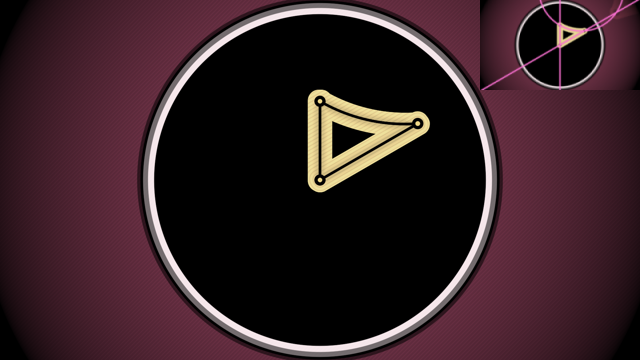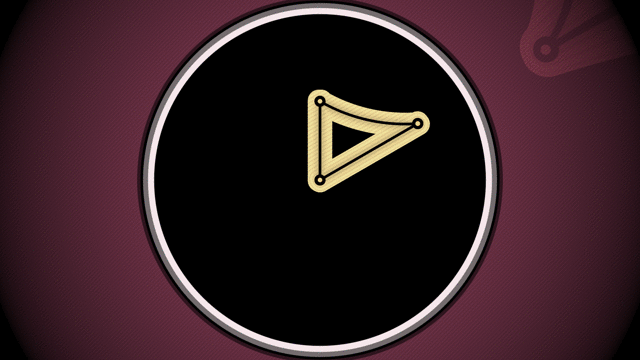紧 (有限顶点) 仿紧 (理想顶点) 非紧 (超理想顶点)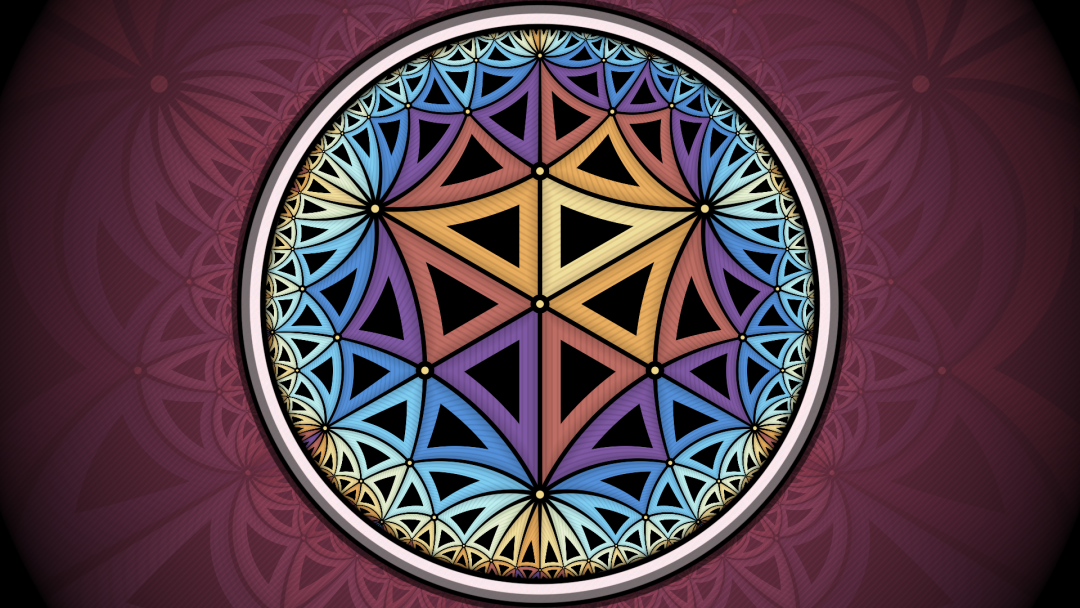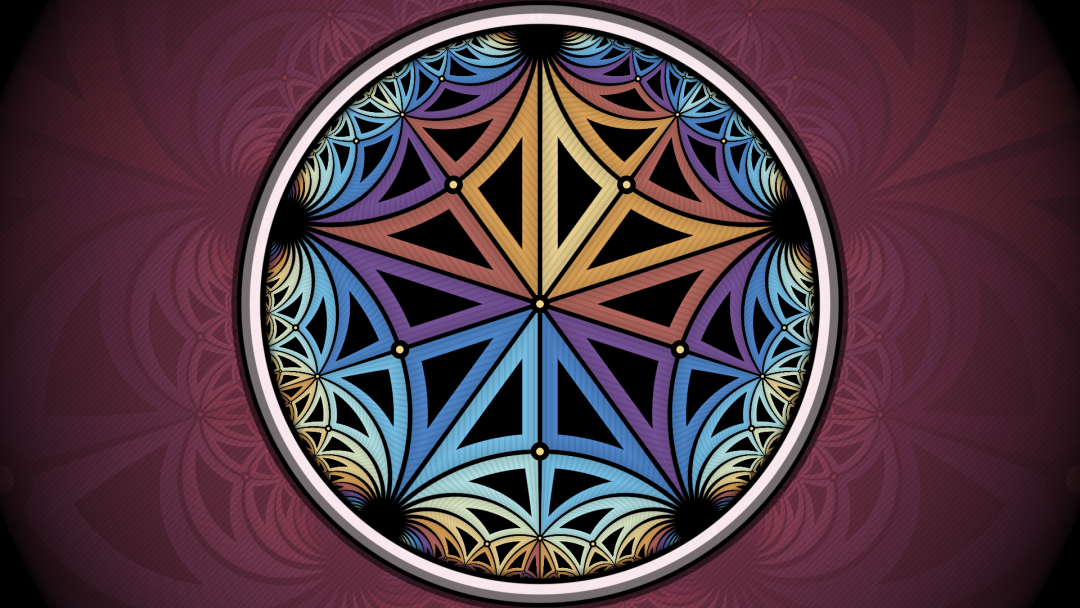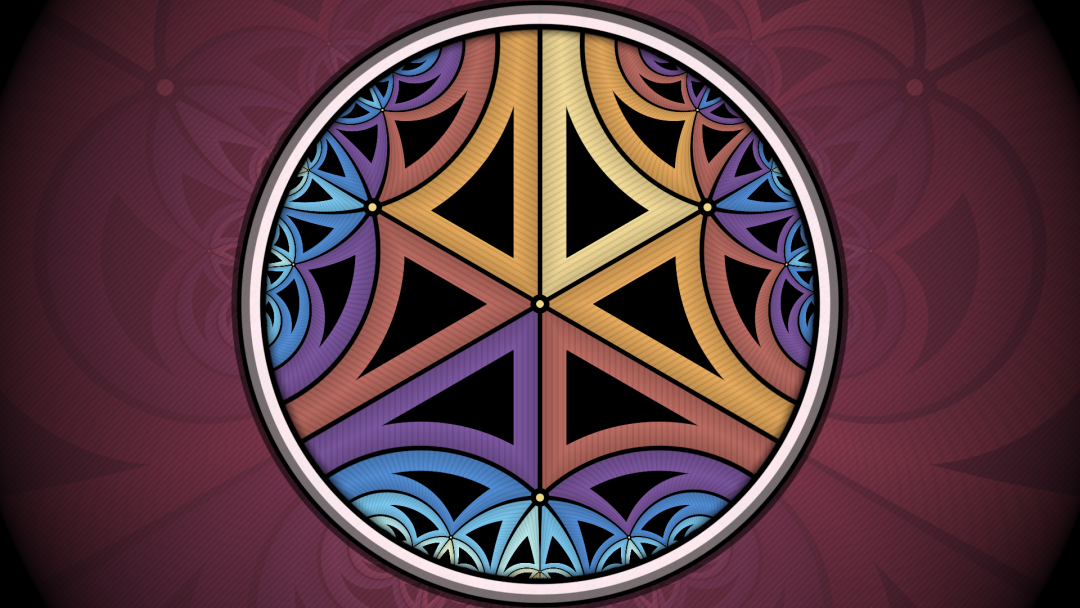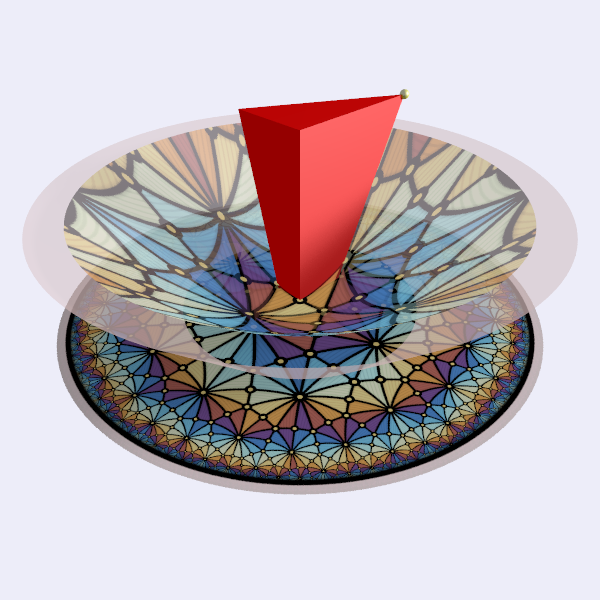1. $P$ 是有限的 (位于空间内部) 当且仅当这个低一维的万花筒是球面的。
2. $P$ 是理想的 (位于空间边界) 当且仅当这个低一维的万花筒是仿射的。
3. $P$ 是超理想的 (位于空间外部) 当且仅当这个低一维的万花筒是双曲的。

# 三维双曲蜂巢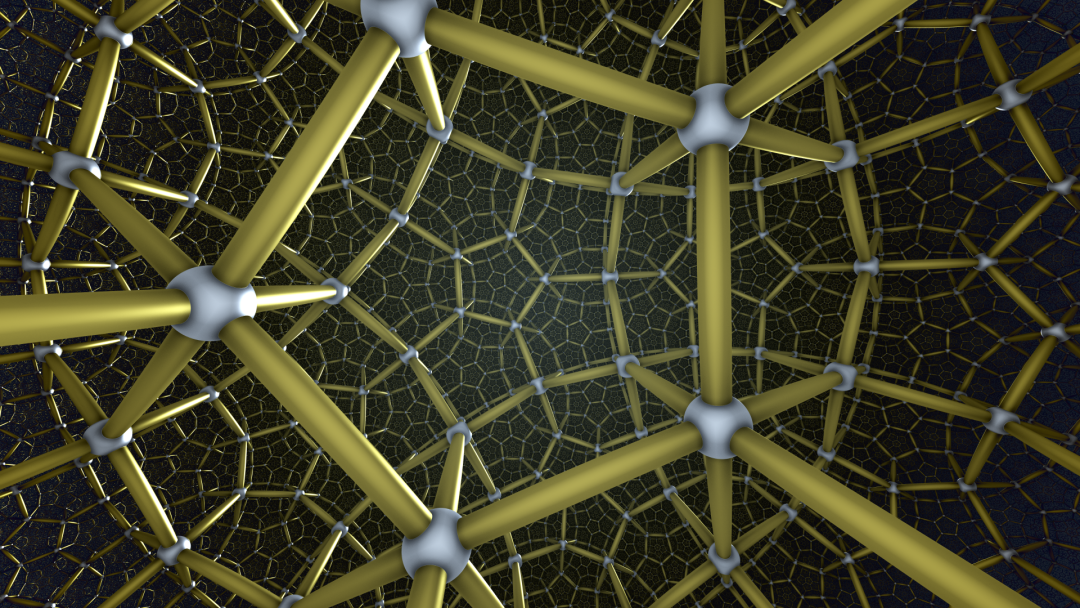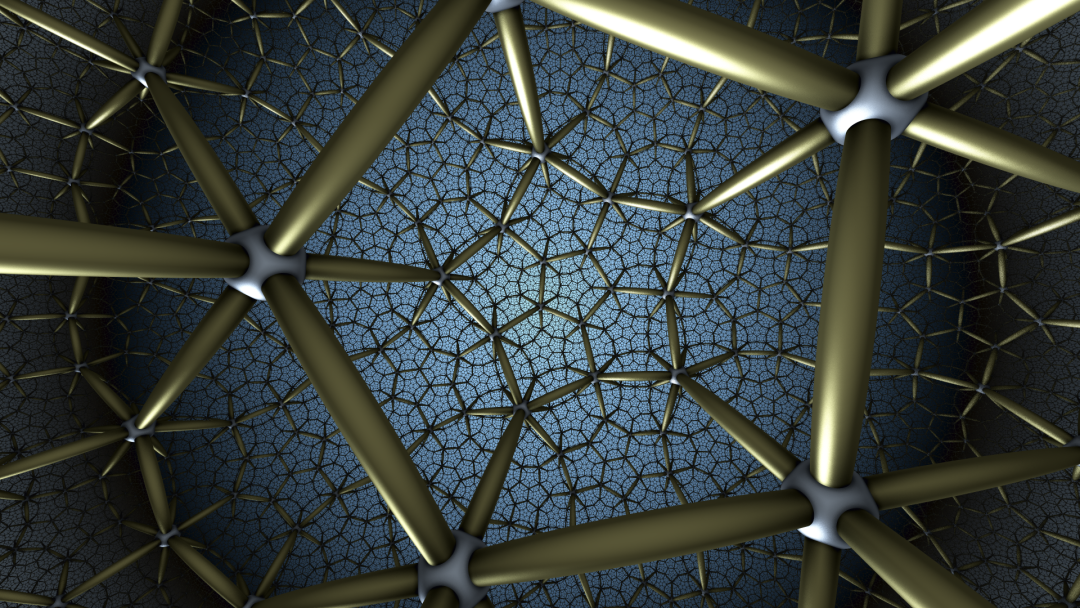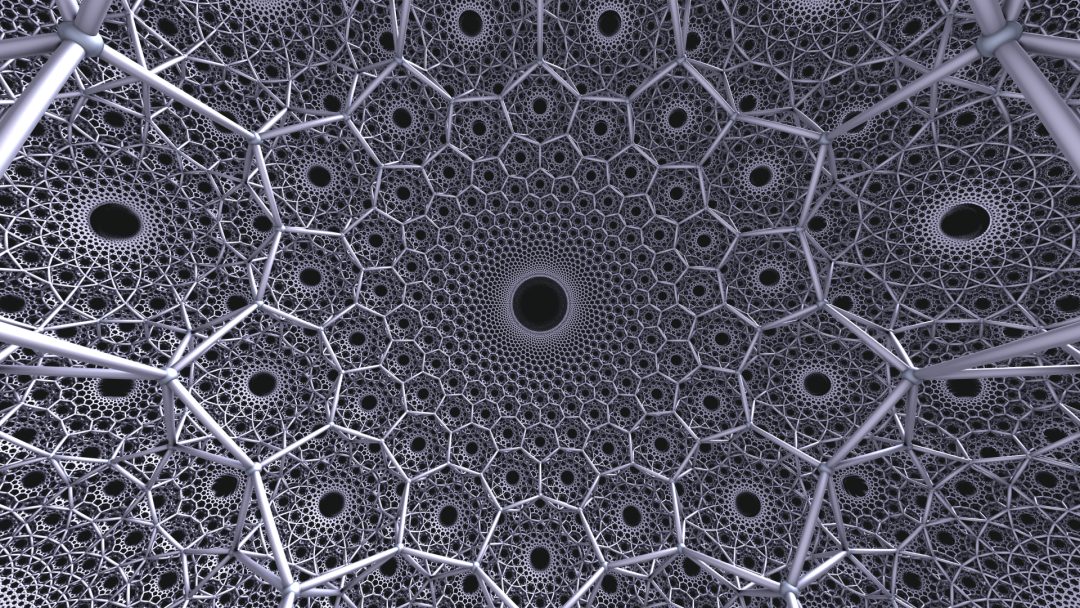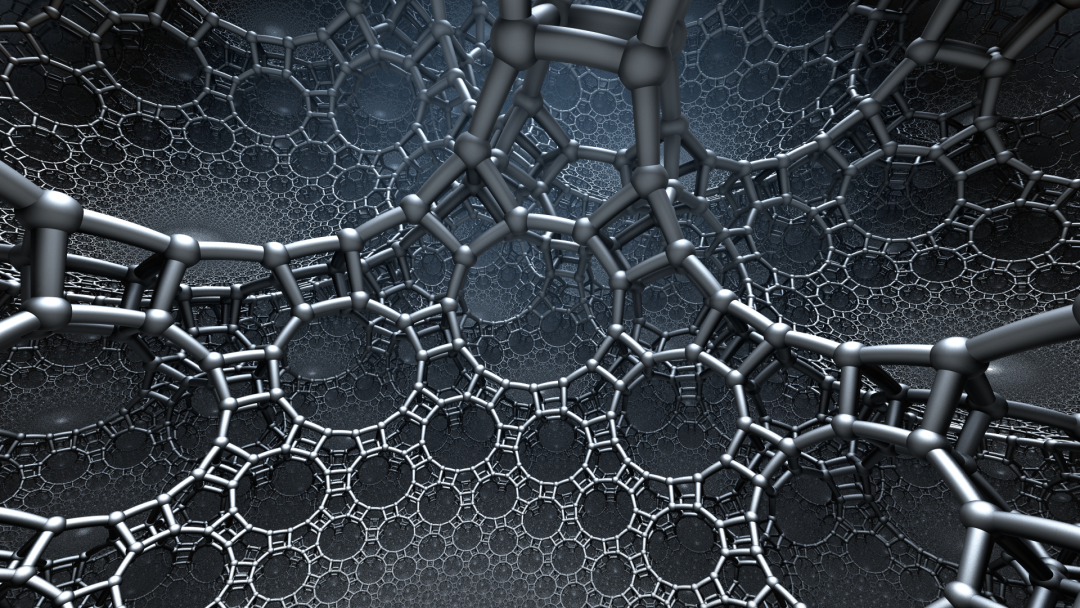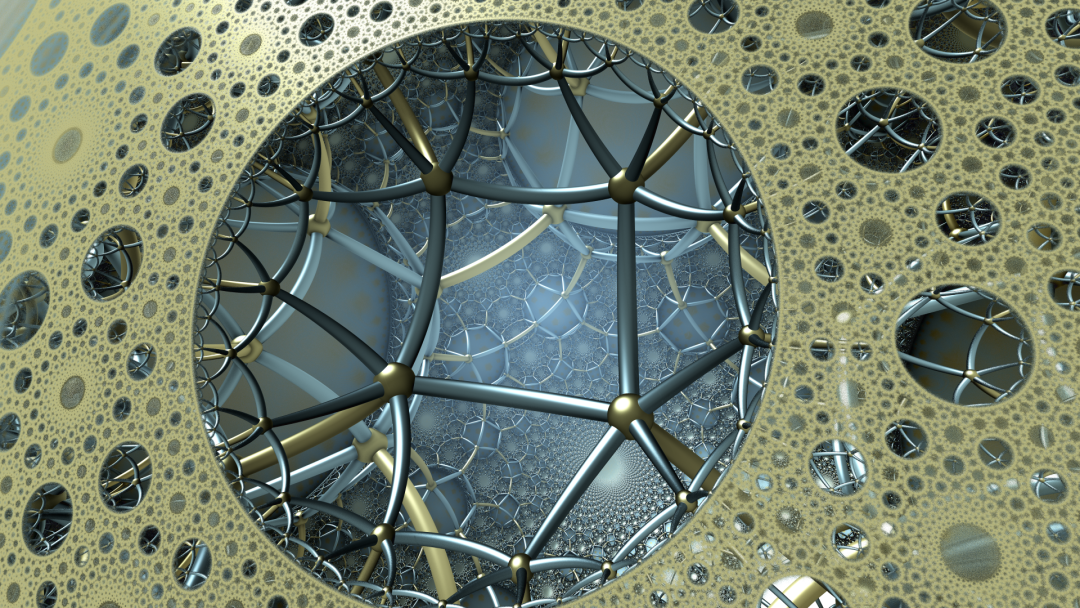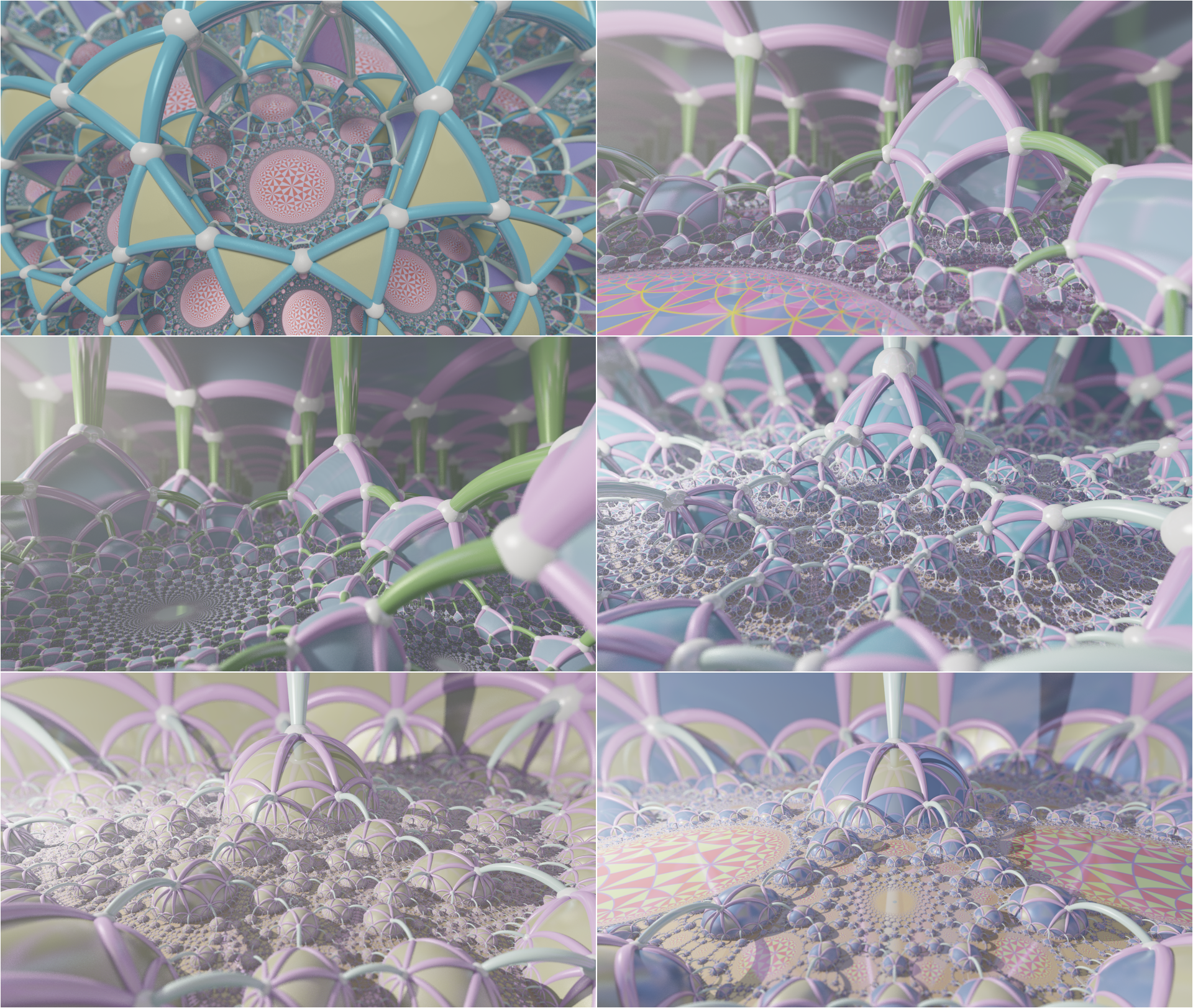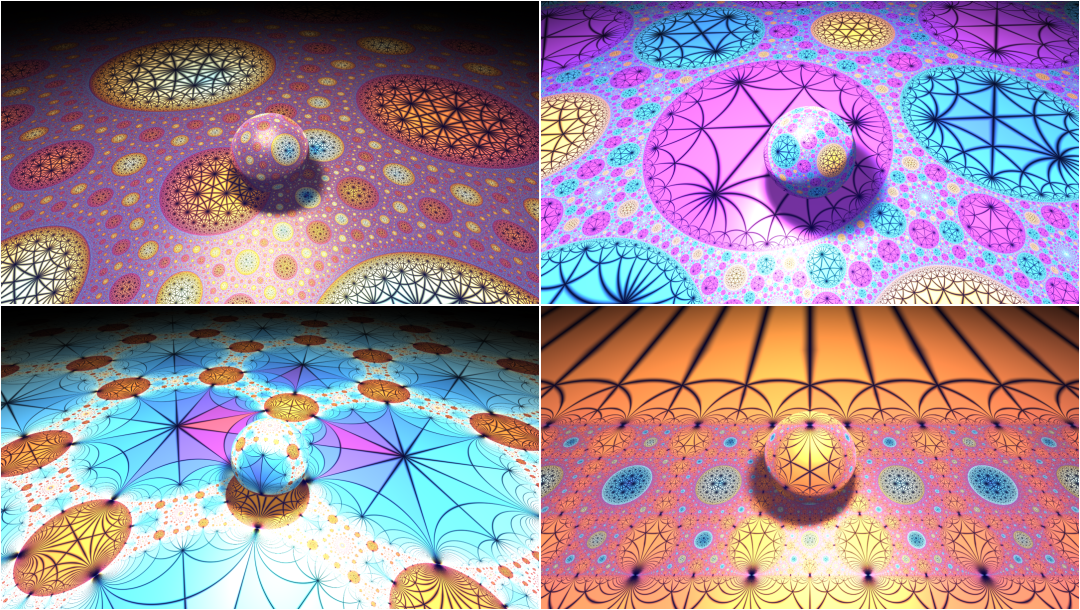# 一沙一世界，一叶一菩提

1. 二维的情形单位圆内部是蜂巢，边界上出现了无数弧段拼成的“arc packing”，而单位圆可以在球极投影下等同于 $\mathbb{R}^1\cup\{\infty\}$
2. 三维的情形单位球内部是蜂巢，边界上是圆堆，而单位球面可以在球极投影下等同于 $\mathbb{R}^2\cup\{\infty\}$，所以我们得到一个二维欧氏平面的圆堆。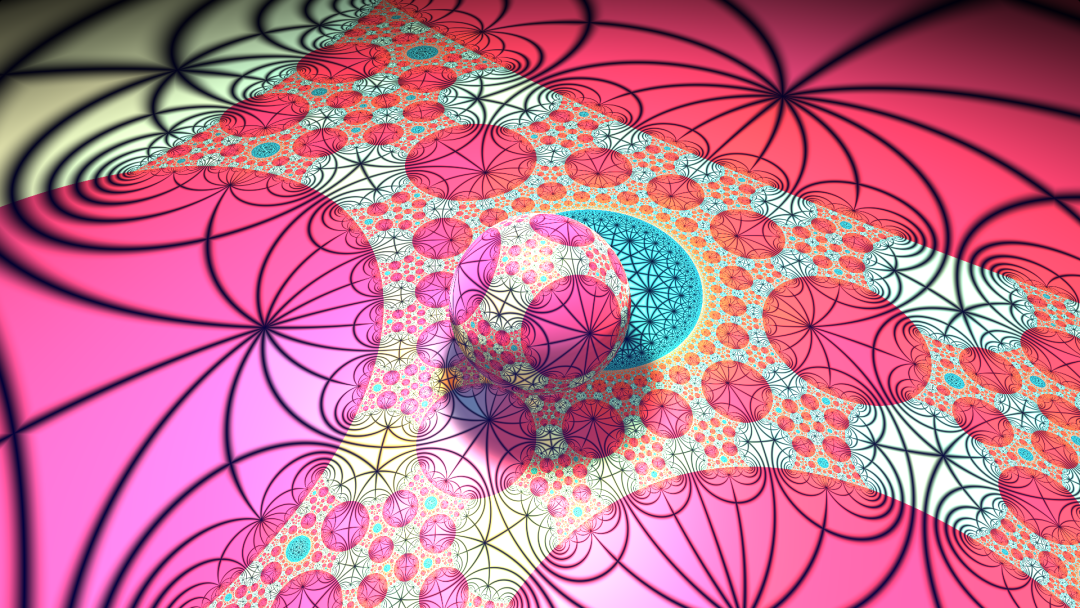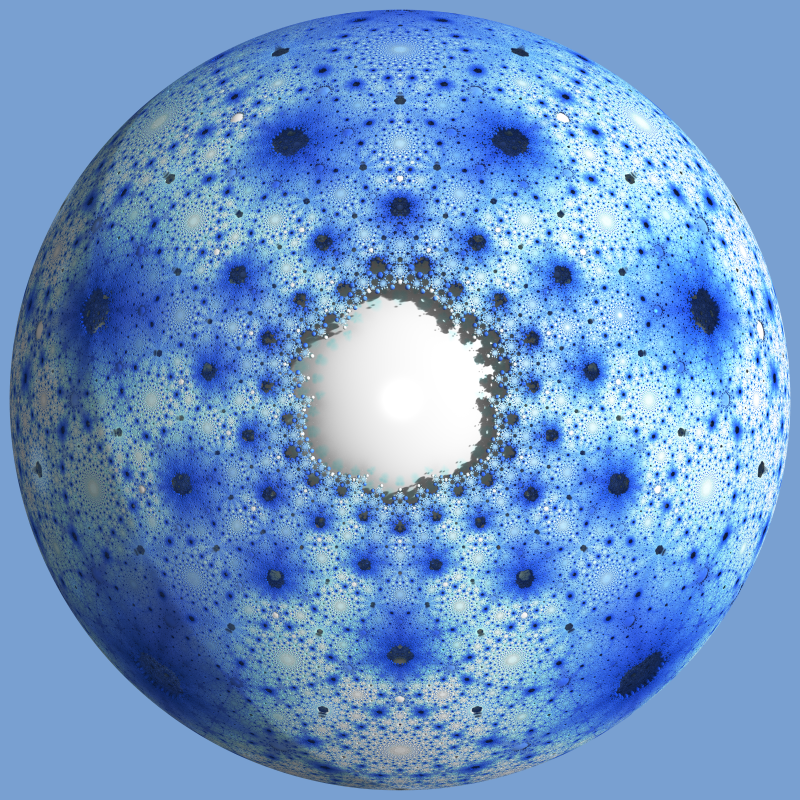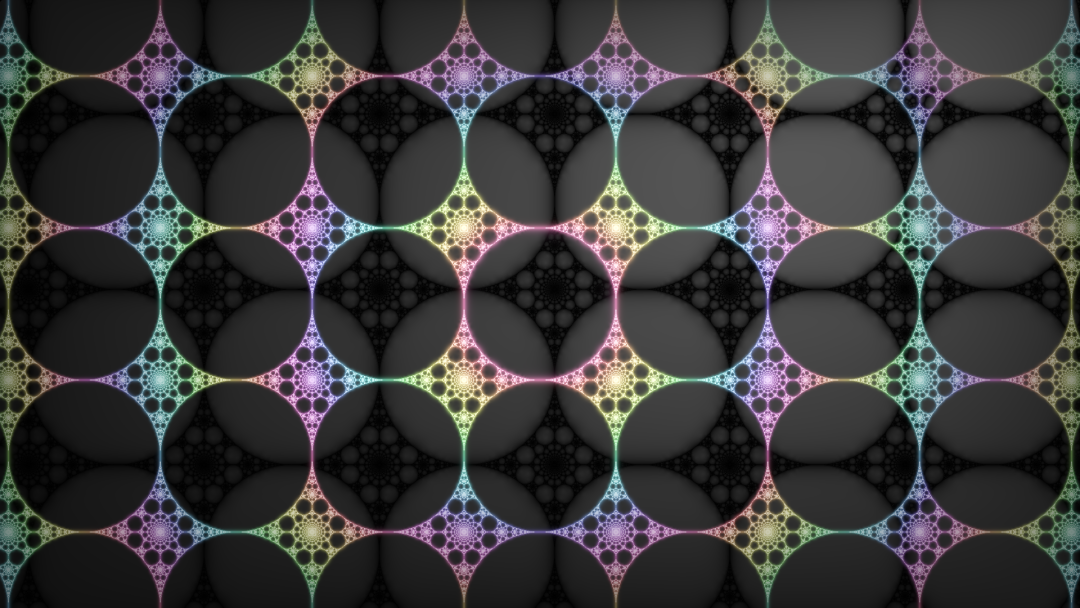rank=5, level=3 rank=5, level=4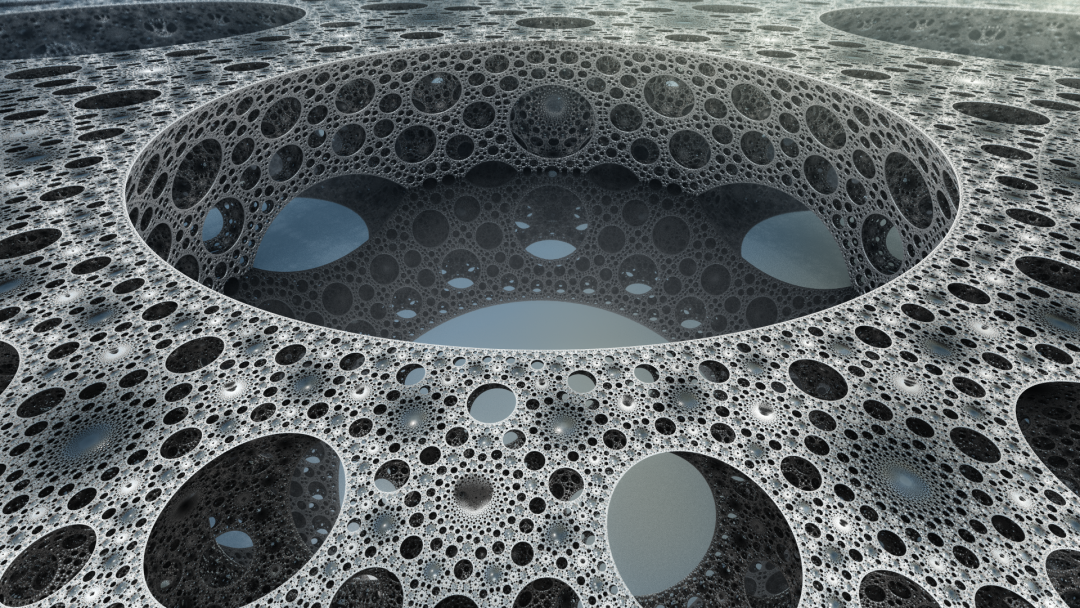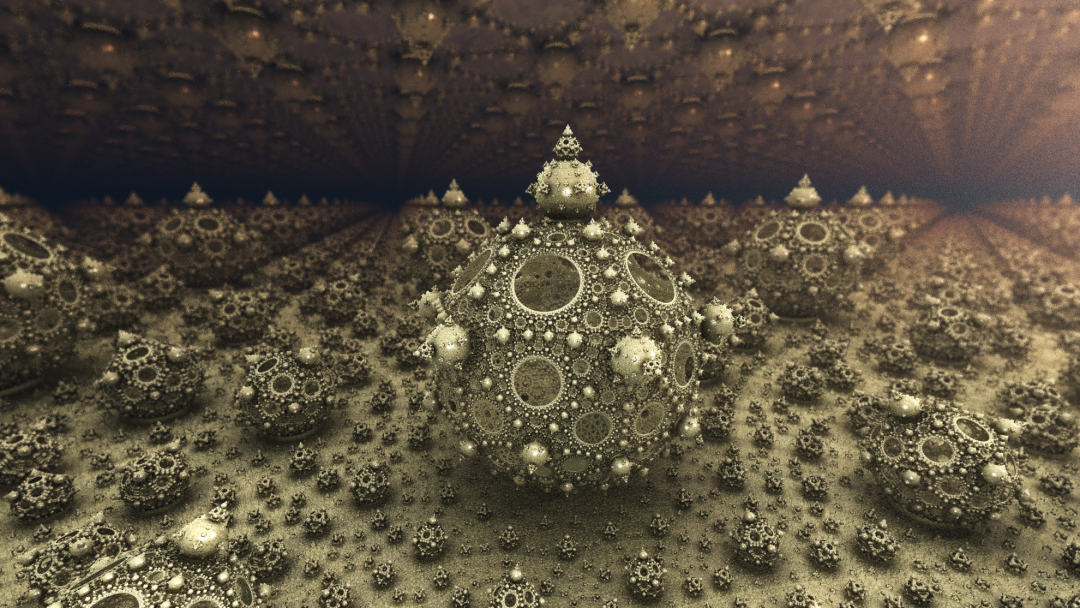# 关于这个项目

knighty 应该是最早提出使用 raymarching 技巧渲染双曲蜂巢的人。他在旧版的分形论坛 www.fractalforums.com 上公开了这一方法。本项目中渲染三维蜂巢的程序就是基于他的方法。此外 knighty 也是所谓 "pseudo Kleinian" 分形的发现者，你可以在网络上搜索到大量关于这一分形的精美图片。在与 knighty 的私信讨论中他耐心地向我解释了 raymarching 技巧中的许多细节，并对项目提出了很多有用的修改意见。

# 附录：技术细节补充

## Coxeter 群必须是有限、仿射或者双曲的吗?

$M=(m_{ij})_{1\leq i,j\leq n}$ 是 Coxeter 矩阵，$C = \left(-\cos\dfrac{\pi}{m_{ij}}\right)_{1\leq i,j\leq n}$ 叫做 Cartan 矩阵，其中当 $M$ 中的某个元素是 Vinberg 记号下的实数 $a$ 时，Cartan 矩阵中对应的元素用 $a$ 代替。我们假设 Coxeter 群是不可约的，即其 Coxeter-Dynkin 图是连通的，这时有如下判定：

1. Coxeter 群是有限的当且仅当 $C$ 正定。
2. Coxeter 群是仿射的当且仅当 $C$ 的符号为 $(n-1, 0)$
3. Coxeter 群是双曲的当且仅当 $C$ 的符号为 $(n-1, 1)$

Maxwell 证明了 level = 2 的群必然是双曲的，对 level >=3 的群则不一定。

## 反演变换作为高维空间中的线性变换

$\mathbf{x}\in\mathbb{R^n}$ 是欧氏空间中一点，为了方便我们取南极点 $(0,0,\ldots,-1)$ 为球极投影中的极点，在逆球极投影下它的像为 $\mathbf{X} = \left(\frac{2\mathbf{x}}{1+\mathbf{x}^2}, \frac{1-\mathbf{x}^2}{1+ \mathbf{x}^2}\right)\in S^n\subset\mathbb{R}^{n+1}.$ 这里我用记号 $\mathbf{x}^2$ 来表示欧氏内积 $\mathbf{x}\cdot\mathbf{x}$

1. $\mathbb{R}^n$ 中以一点 $\mathbf{p}$ 为中心，$r>0$ 为半径的球可以表示为 $(\mathbf{x-p})^2\leq r^2.$ 直接验证可得这等价于 $\langle\mathbf{\xi}, \mathbf{\omega}\rangle_L \geq 0$，其中 $\mathbf{\omega} = \left(\frac{\mathbf{p}}{r}, \frac{1-\mathbf{p}^2+r^2}{2r}, \frac{1+\mathbf{p}^2-r^2}{2r}\right).$$\langle\cdot,\cdot\rangle_L$$\mathbb{R}^{n+1,1}$ 中的 Lorentz 内积： $\langle \mathbf{x},\mathbf{y} \rangle_L = \sum_{i=1}^n x_iy_i - x_{n+1}y_{n+1}.$ 注意到 $\mathbf{\omega}$ 的分母上都除以 $r$，这是为了使得 $\langle \mathbf{\omega},\mathbf{\omega} \rangle_L = 1$，即 $\mathbf{\omega}$ 是 Lorentz 内积下的单位向量，并且是 space-like 的。

球的外部 $(\mathbf{x-p})^2\geq r^2$ 自然可以表示为 $\langle\mathbf{\xi}, \mathbf{\omega}\rangle_L \leq 0$。所以欧氏空间中的球面确实可以表示为高维双曲空间中的半空间。

2. $\mathbb{R}^n$ 中以 $\mathbf{n}$ 为单位法向量，与原点距离为 $d$ 的超平面的正半空间可以表示为 $\mathbf{x}\cdot\mathbf{n}\geq d.$ 直接验证可得这等价于 $\langle\mathbf{\xi}, \mathbf{\omega}\rangle_L \geq 0$，其中 $\mathbf{\omega} = (\mathbf{n}, -d, d)$$\mathbf{\omega}$ 仍然是 Lorentz 内积下 space-like 的单位向量。

$\mathbf{\omega}_1,\mathbf{\omega}_2\in\mathbb{R}^{n+1,1}$ 分别对应 $\mathbb{R}^n$ 中的两个球 $(\mathbf{p}_1,r_1)$$(\mathbf{p}_2,r_2)$，则 $\langle \mathbf{\omega}_1,\mathbf{\omega}_2\rangle_L=\frac{r_1^2+r_2^2-|\mathbf{p_1-p_2}|^2}{2r_1r_2}=\pm1 + \frac{(r_1^2\mp r_2^2)-|\mathbf{p_1-p_2}|^2}{2r_1r_2}.$ 于是 $\langle \mathbf{\omega}_1,\mathbf{\omega}_2\rangle_L\in[-1, 1]$ 当且仅当 $|r_1-r_2| \leq |\mathbf{p_1-p_2}|\leq r_1+r_2,$ 即两球有交点。且等于 $-1$ 时两球外切，等于 $+1$ 时两球内切。

## 超理想顶点的进一步解释

 Poincaré 模型 Klein 模型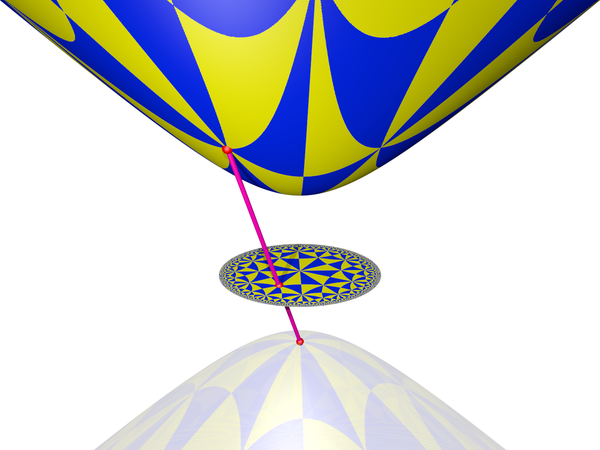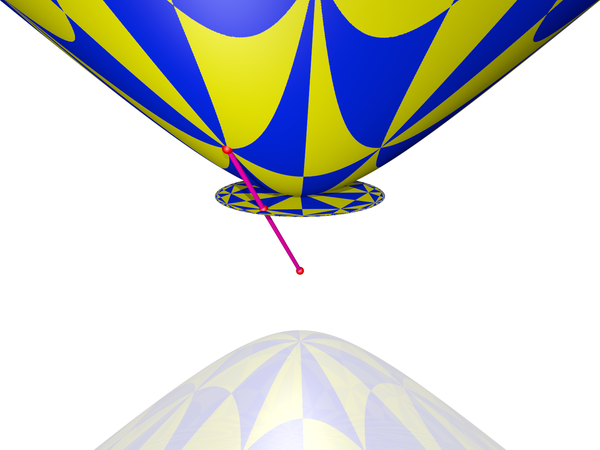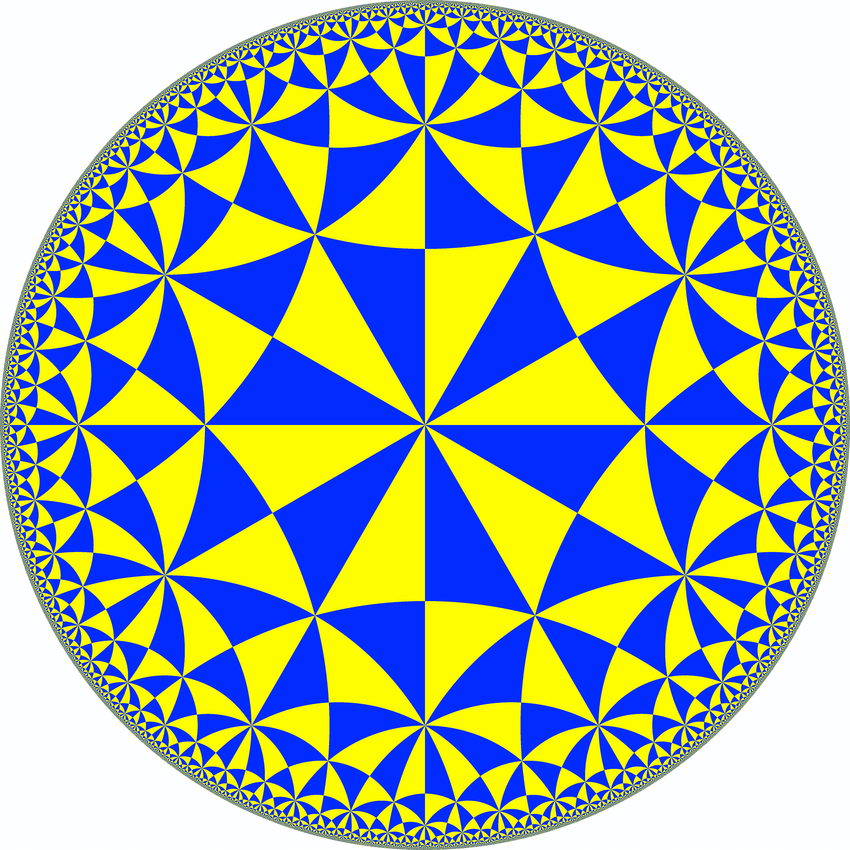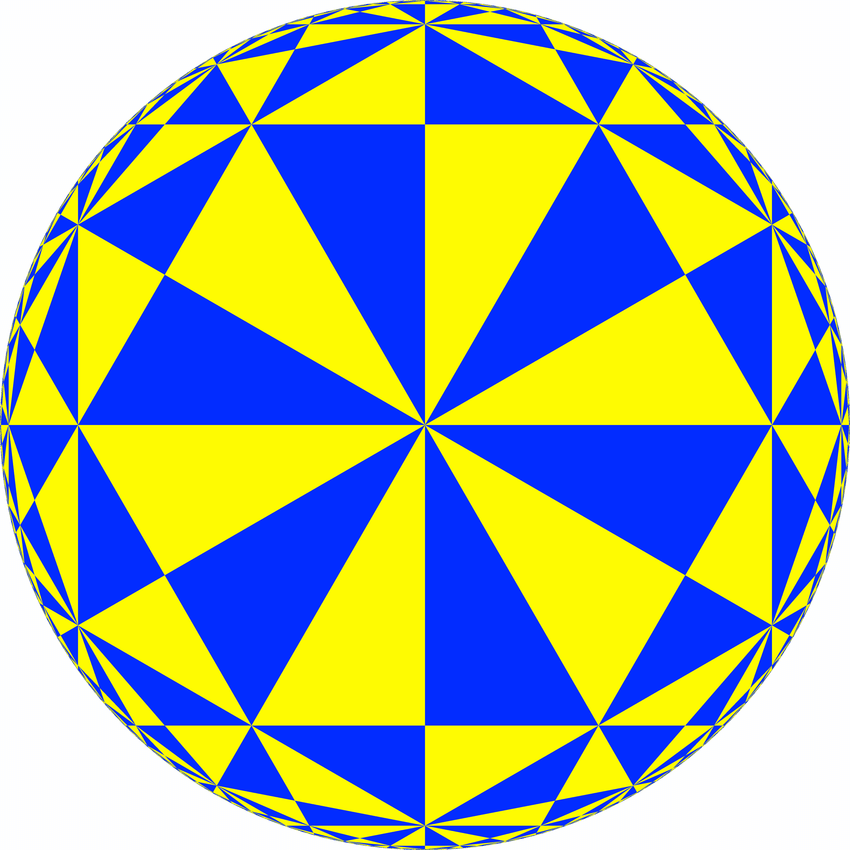Klein 模型与 Poincaré 模型类似，都来自双曲空间在高一维空间的投影，区别在于投影选择的极点不同，所以所处的位置也不同。Poincaré 空间位于过原点的平面 $x_{n+1}=0$ 上，而 Klein 模型位于与双曲抛物面相切的平面 $x_{n+1}=1$ 上。过原点的超平面与双曲抛物面的交线投影到 Poincaré 模型后则变为与单位圆正交的测地线，而投影到 Klein 模型则以后变为直线。

1. $\xi$ 位于光锥内部，所以是 time-like 的：$\langle\xi,\xi\rangle_L<0$，内积限制在 $\xi^\bot$ 上是正定的，$\xi^\bot$ 与光锥没有非零交点。

2. $\xi$ 位于光锥边界，所以是 light-like 的：$\langle\xi,\xi\rangle_L=0$，内积限制在 $\xi^\bot$ 上的 Sylvester 符号为 $(n-1, 0)$$\xi^\bot$ 与光锥相切于 $\xi$ 5

3. $\xi$ 位于光锥外部，所以是 space-like 的：$\langle\xi,\xi\rangle_L>0$，内积限制在 $\xi^\bot$ 上符号为 $(n-1,1)$$\xi^\bot$ 与光锥之交是一个球帽 (sphere cap)。# 参考文献

1. G. Maxwell. Sphere packings and hyperbolic reflection groups. J. Algebra 79 (1982), no. 1, 78–97.
2. È. B. Vinberg. Discrete groups generated by reflections.
3. Hao Chen, Jean-Philippe Labbé. Lorentzian Coxeter systems and Boyd-Maxwell ball packings.
4. Thomas E. Cecil, Lie Sphere Geometry, 2nd ed., Universitext, Springer, New York, 2008.
5. John G. Ratcliffe. Foundations of Hyperbolic Manifolds. Graduate Texts in Mathematics book series (GTM, volume 149).
6. Roice Nelson, Henry Segerman. Visualizing Hyperbolic Honeycombs.

1. 这段材料要感谢陈浩老师推荐。↩︎

2. 这里我们暂时先不考虑 Vinberg 记号的情形，见后文。↩︎

3. 注意到每个万花筒都使用了四面镜子，所以 up 主应该是取两个互相垂直的镜子 $m_1,m_2$ 过原点，然后将镜子 $m_3$ 关于 $m_1,m_2$ 反复反射。$m_3$$m_1,m_2$ 生成的 dihedral 群作用下有四个像，正好围成一个圆周，这就是视频中的四面镜子。然后拿走镜子 $m_1,m_2$，把塑料棒放在镜子 $m_1$ 或者 $m_2$ 的位置上，使得塑料棒中间部分位于原点，这相当于使得塑料棒首先关于 $m_2$ (或 $m_1$) 成像一次，得到的就是正多面体。↩︎

4. 这个蜂巢的顶点实际上位于一个 horosphere 上：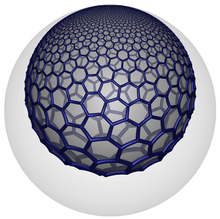这个 horosphere 可以通过一个反演变换变成欧式空间的一个正六边形密铺。↩︎

5. 见参考文献中 Ratcliffe 的书定理 3.1.1。↩︎

|